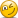Stulz reading - Investors & Risk Mgmt

[email protected]

New Member
Hi David,
In AIM asking for hedging price risk with respect to output which wont affect firm value (Stulz Chap 2), if u can explain how arbitrage & hedging is used.
I feel I have lost touch with beta implicationsI dint understand +ve/-ve beta & long/short position!
If u can give some example.
Thanks.
~Anil

David Harper CFA FRM

David Harper CFA FRM
Staff member
Subscriber
Hi Anil,

On the member page is a small spreadsheet that I hope will help explain this.
See 2008 OpRisk: Stulz’ Hedging Price Risk.

I think Stulz 2 & 3 are incredibly hard to read.
Chapter 2 is (basically) the theoretical idea that risk management cannot add value.
Chapter 3 is (basically) the practical exceptions that allow for risk management to add value.

A good "analogy" IMO is one of their own examples: the theory of Modigliani-Miller irrelevance proposition is "capital structure does not matter" under unrealistic assumptions. In practice, the unrealistic assumptions do not apply, so capital structure does matter. So, Chapter 2 is: reducing neither systematic nor idiosyncratic risk creates value. Chapter 3 is: due to practical frictions, Chapter 2 is incorrect.

Under hedging price risk, please note the example in the XLS is the same as Stulz' Pure Gold, Inc. There are two scenarios:

Unhedged firm: PV = $360/(1+riskless rate + beta*ERP) =$333
Hedged firm: PV = $350/(1+riskless rate) =$333

The key idea here is essentially similar to the idea is risk-neutral valuation; how can a risky asset (i.e., an asset with systematic risk) have the same price (PV) as a riskless asset?
Answer: The higher expected future price (note: higher systematic risk implies higher return per CAPM) will also use a higher discount rate; the higher FV will be offset by the higher discount rate so that the PV is the same!

In the XLS, it is really just:

hedged = 350/(1+5% riskless) and un-hedged = 360/(1+8%) = \$333 PV

Regards hedged, the firm/asset is riskless, so beta = 0 and (i) lower expected FV, but (ii) discount at riskless
Regards unhedged, the firm/asset has systematic risk, so there is a beta. But the beta is both (i) increasing the future value (FV) and also (ii) increasing the discount rate.

David